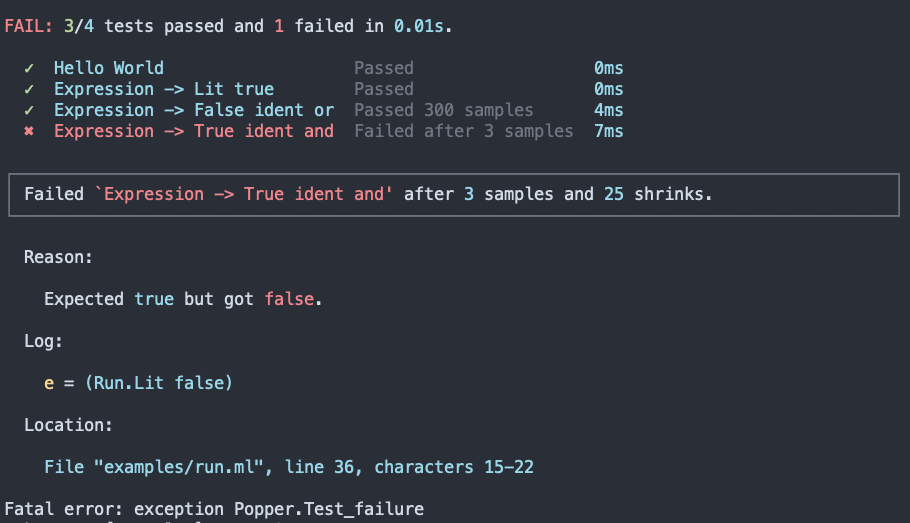Popper is an OCaml testing library that can be used for writing simple unit-tests as well as property-based ones. Its underlying design is inspired by the Python library Hypothesis.

Warning

Popper is currently in alpha-stage and is to be used at your own risk.

High-level features of Popper include:

• A uniform API for defining regular unit- and property-based tests.
• Embedded shrinking — invariants used when constructing samples for property-based tests are always respected.
• Compositional design — tests may be bundled and nested arbitrarily.
• Ships with a `ppx` for automatically deriving comparator and sample functions for custom data types.
• Deterministic (and reproducible) results.
• Colorful output (cred goes to Alcotest, couldn't resist the inspiration).
• Support for line-number reporting, timing information and logging.

## Show me an example

Here's what test output looks like:It was generated from the following code:

``````open Popper
open Sample.Syntax

type exp =
| Lit of bool
| And of exp * exp
| Or of exp * exp
| Not of exp
[@@deriving show, ord, popper]

(* A buggy evaluator function *)
let rec eval = function
| Lit b -> b
| And (e1, e2) -> eval e1 || eval e2
| Or (e1, e2) -> eval e1 || eval e2
| Not b -> not @@ eval b

(* A simple unit test *)
let test_hello_world =
test @@ fun () ->
equal Comparator.string "hello world" (String.lowercase_ascii "Hello World")

(* Another unit test *)
let test_lit_true = test @@ fun () -> is_true (eval (Lit true) = true)

(* A property-based test *)
let test_false_ident_or =
test @@ fun () ->
let* e = exp_sample in
is_true (eval e = eval (Or (Lit false, e)))

(* Another property-based test *)
let test_true_ident_and =
test @@ fun () ->
let* e = Sample.with_log "e" pp_exp exp_sample in
is_true ~loc:__LOC__ (eval e = eval (And (Lit true, e)))

(* Bundle some tests together *)
let exp_suite =
suite
[ ("Lit true", test_lit_true)
; ("False ident or", test_false_ident_or)
; ("True ident and", test_true_ident_and)
]

(* Top-level test-suite *)
let suite =
suite [ ("Hello World", test_hello_world); ("Expression", exp_suite) ]

let () = run suite
``````